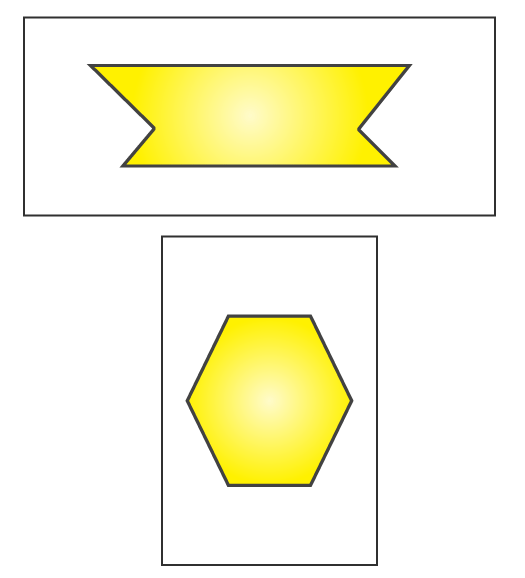## Access NCERT Solutions for Class 6 Maths Chapter 13 Symmetry Exercise 13.2

### Access NCERT Solutions for Class 6 Maths Chapter 13 Symmetry Exercise 13.2

1. Find the number of lines of symmetry for each of the following shapes :Solutions:

(a) For the given figure there are 4 lines of symmetry.

(b) For the given figure there are 4 lines of symmetry.

(c) For the given figure there are 4 lines of symmetry.

(d) For the given figure there is only 1 line of symmetry.

(e) For the given figure there are 6 lines of symmetry.

(f) For the given figure there are 6 lines of symmetry.

(g) For the given figure there is no line of symmetry.

(h) For the given figure there is no line of symmetry.

(i) For the given figure there are 3 lines of symmetry.

Now we may observe the lines of symmetry in the above figures as follows2. Copy the triangle in each of the following figures on squared paper. In each case, draw the line(s) of symmetry, if any and identify the type of triangle. (Some of you may like to trace the figures and try paper-folding first!)Solutions:

(a) The given triangle is an isosceles triangle. Here it will be only 1 line of symmetry.(b) The given triangle is an isosceles triangle. Here it will be only 1 line of symmetry.(c) The given triangle is a right angled triangle. Here it will be only 1 line of symmetry.(d) The given triangle is a scalene triangle. Here it will be no line of symmetry.

3. Complete the following table.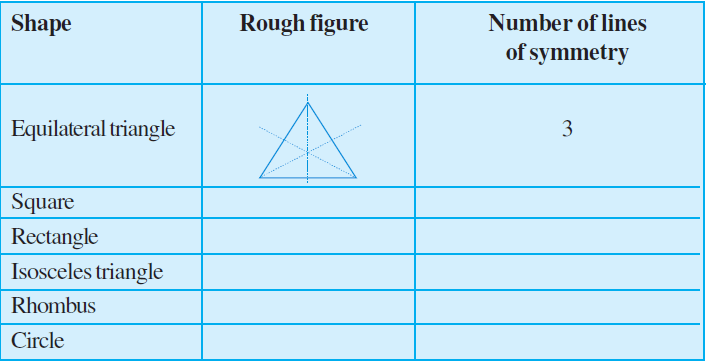Solutions:

The given table is completed as follows: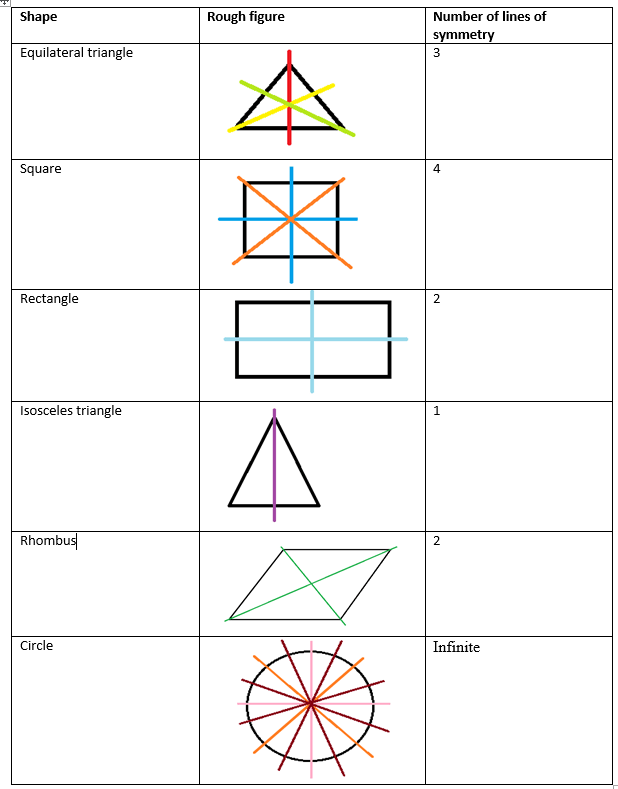In case of circles we find infinite lines. Here some lines of symmetry are drawn. Similarly, we can draw more symmetric lines for the circle.

4. Can you draw a triangle which has

(a) exactly one line of symmetry?

(b) exactly two lines of symmetry?

(c) exactly three lines of symmetry?

(d) no lines of symmetry?

Sketch a rough figure in each case.

Solutions:

(a) Yes, we can draw an isosceles triangle which has only 1 line of symmetry.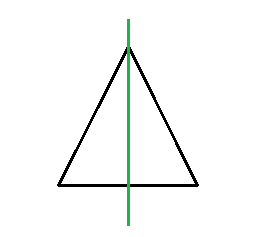(b) No, we cannot draw a triangle which has 2 lines of symmetry.

(c) Yes, we can draw an equilateral triangle which has 3 lines of symmetry.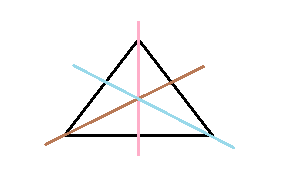(d) Yes, we can draw a scalene triangle which has no line of symmetry.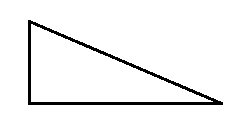5. On a squared paper, sketch the following:

(a) A triangle with a horizontal line of symmetry but no vertical line of symmetry.

(b) A quadrilateral with both horizontal and vertical lines of symmetry.

(c) A quadrilateral with a horizontal line of symmetry but no vertical line of symmetry.

(d) A hexagon with exactly two lines of symmetry.

(e) A hexagon with six lines of symmetry.

Solutions:

(a) A triangle with a horizontal line of symmetry but no vertical line of symmetry can be drawn as follows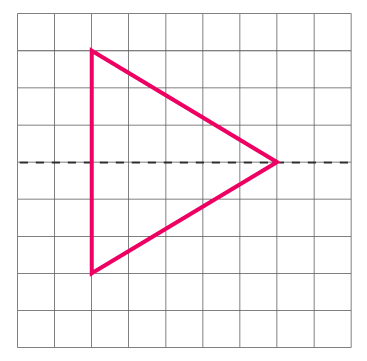(b) A quadrilateral with both horizontal and vertical lines of symmetry can be drawn as follows(c) A quadrilateral with a horizontal line of symmetry but no vertical line of symmetry can be sketched as follows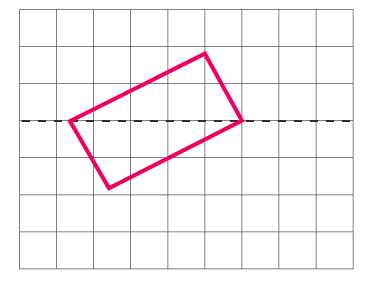(d) A hexagon with exactly two lines of symmetry may be drawn as follows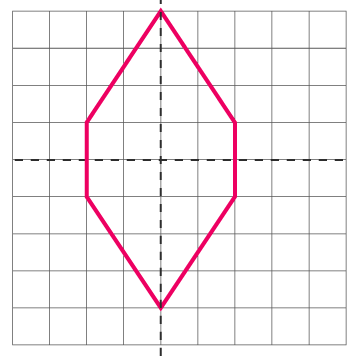(e) A hexagon with 6 lines of symmetry may be drawn as follows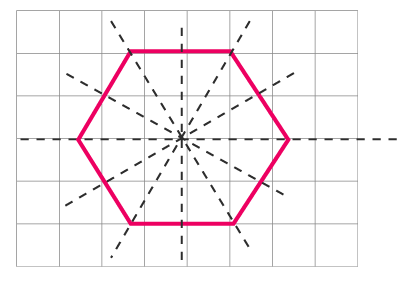6. Trace each figure and draw the lines of symmetry, if any: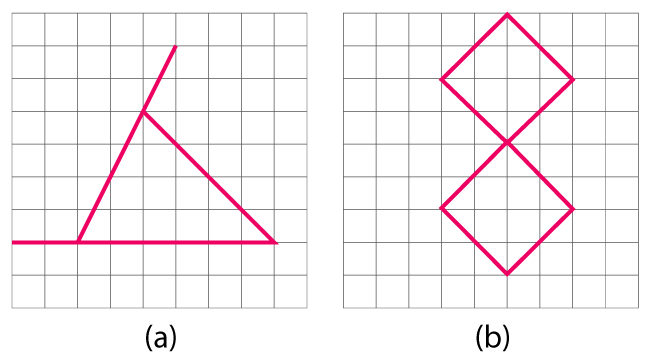Solutions:

(a) The given figure is an isosceles triangle. Hence, there will be 1 line of symmetry.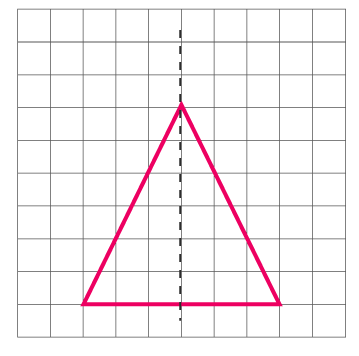(b) The given figure has two lines of symmetry.(c) The given figure has 4 lines of symmetry.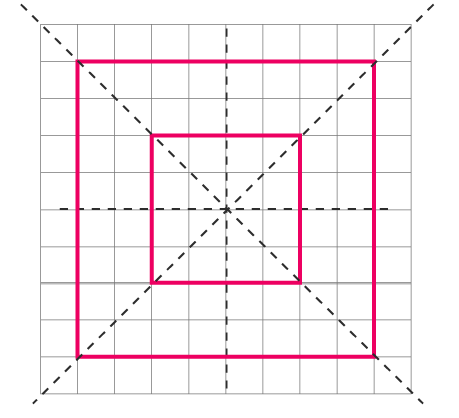(d) The given figure is an octagonal which has 2 lines of symmetry.(e) The given figure has only 1 line of symmetry.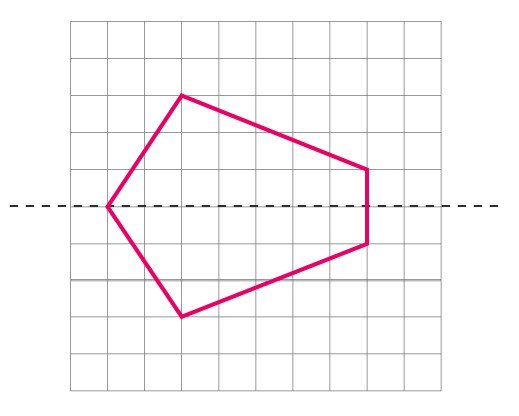(f) The given figure has 4 lines of symmetry.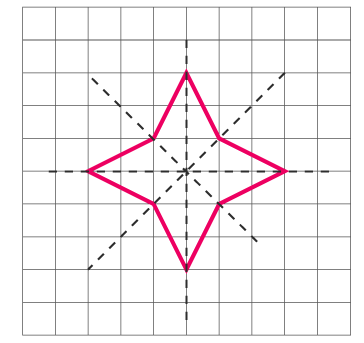7. Consider the letters of English alphabets, A to Z. List among them the letters which have

(a) vertical lines of symmetry (like A)

(b) horizontal lines of symmetry (like B)

(c) no lines of symmetry (like Q)

Solutions:

(a) Vertical lines of symmetry are A, H, I, M, O, T, U, V, W, X, Y.

(b) Horizontal lines of symmetry are B, C, D, E, H, I, K, O, X.

(c) No lines of symmetry are F, G, J, L, N, P, Q, R, S, Z.

8. Given here are figures of a few folded sheets and designs drawn about the fold. In each case, draw a rough diagram of the complete figure that would be seen when the design is cut off.Solutions:

We may draw a diagram of complete figure as follows